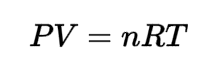Request a Tool

Ideal Gas Law Calculator

To calculate temperature, pressure,volume and moles of gases consumed or produced.

Temperture
0

Pressure
0

Mole of Gas (n)
0

Volume (V)
0

Formula• P = Pressure of the gas
• V = Volume
• n = moles of gas
• T = Temperature
• R = 8.314 J K-1 mol-1, ideal gas constant.

Defination / Uses

The ideal gas law is the equation of state of a hypothetical ideal gas. It is a good approximation of the behaviour of many gases under many conditions, although it has several limitations.

Uses

ideal gas law can be used to calculate Temperature, Pressure,Volume and Moles of gases consumed or produced.

This ideal gas law calculator can help to determine the parameters of an ideal gas as a result of changes in pressure, temperature, or volume. Continue reading to learn about the properties of an ideal gas, how to utilise the ideal gas law equation, and how to calculate the ideal gas constant. An ideal gas is a hypothetical gas made up by scientists and students because the Ideal Gas Law would be lot simpler if things like intermolecular forces didn't exist to complicate it. Ideal gases are simply point masses travelling in a straight line at a constant, random speed. The assumptions given in the Kinetic-Molecular Theory of Gases characterise its behaviour. Use the upper given formula for manual calculations. No sign-up, registration OR captcha is required to use this tool.